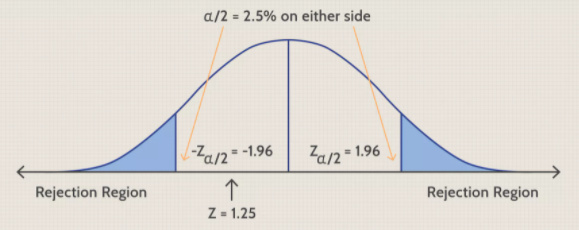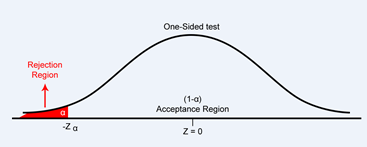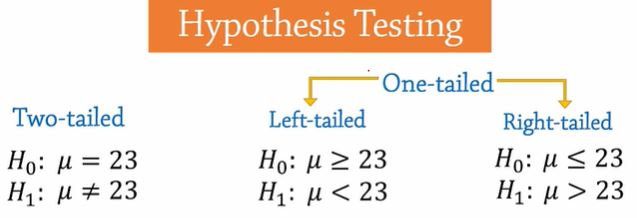# Introduction to Hypothesis Testing

Hello Everyone

In this Blog, I’m going to briefly go over the essentials of hypothesis testing.

A hypothesis is simply a claim or statement about a population parameter such as the population mean, variance, proportion, and so on. The beauty of the Hypothesis is that it can be TESTED!

(Nightmare: Hypothesis Test)

In inferential statistics, we do not work on entire population instead we work on a sample or samples, and use the results to generalize on an entire population or populations.

Suppose we make a claim that the average age of all college students in a city is 23, which we write as: μ = 23

Since this hypothesis contains equality, we refer to it as the null hypothesis, represented by Ho.

The complement or opposite of the null is the alternative hypothesis, represented by
Ha or H1.

In this case, the alternative will be that the mean is not equal to 23.(µ ≠ 23)

We use these hypotheses for two- tailed tests because the alternative basically means that
the mean could be less or greater than 23.Suppose instead we want to test if the mean is less than 23. Since this statement does not contain equality, we will assign it to the alternative hypothesis. And the null hypothesis will be the opposite. That is, the mean is greater or equal to 23. And if we’re testing if the mean is greater than 23, that will be the alternative hypothesis.And the null will be, mean less or equal to 23. These two are hypotheses for one-tailed tests.

For a left-tailed test the alternative hypothesis has a less than sign; and for a right tailed test the alternate has a greater than sign.

Note that the alternative hypotheses determine the tail of the test.

Note also that the alternative hypotheses never contains equality sign , and the null always

contains equality sign.Now when we conduct hypothesis tests, we’re primarily trying to decide whether or not

to reject the null hypothesis.

If we reject the null hypothesis, we will say “we have enough evidence to support the alternative hypothesis”, or “to say that the alternative hypothesis is true.”

If we do not reject the null hypothesis, we will say that we do not have enough evidence to support the alternative hypothesis.

We usually don’t say that the alternative hypothesis is not true.

We only say that we don’t have enough evidence to support it.

Now, to determine when to reject the null hypothesis, we select a significance level alpha.

5% or 0.05 is the most commonly used alpha value.

0.10 and .01 are also often used.

The significance level helps specify the size of the region where the null hypothesis should be rejected.

We call that region, the critical or rejection region.

For a two-tailed test, alpha is divided by 2 into both tails.(0.025)

So we reject the null hypothesis in the tails and we do not reject it in the middle.

For one tailed-tests, alpha is not divided.

So for the left-tailed test, the rejection region is in the left tail, and it is in the right tail for the right tailed test.

Once the rejection region is set, we then calculate the test statistic.

If the test statistic falls in the rejection region, we reject the null hypothesis.

Otherwise, we fail to reject it.

Stay tuned for the next part of the blog where we will look at an example to illustrate the hypothesis testing process.